Strain Plot PropertyManager

The Strain Plot PropertyManager allows you to plot strain results for static, nonlinear, modal time history and drop test studies.

To display this PropertyManager, run a static, nonlinear, dynamic study, or drop test study. Right-click Results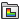and select Define Strain Plot.

Display

Component Select a strain component to plot. Directions are based on the selected reference geometry.

See Strain Components.

Shell Face For shell and mixed mesh models only:
 Top Total strains at the top face Bottom Total strains at the bottom face. Membrane Membrane strain component. Bending Bending strain component.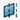Plane, Axis or Coordinate System Select a reference plane, an axis, or a coordinate system to plot strain. Used for directional components only.
Show as vector plot Generates a vector plot where a vector is plotted at each node to show the magnitude and direction of the selected component. You can control the size and density of the vectors in a vector plot using the Vector Plot Options PropertyManager. Available for directional strain components only.
Strain Type For nonlinear studies only, select from the drop-down menu:
 Total Total strain due to various effects. Plastic Non-recoverable strain. Elastic Recoverable strain. Thermal Strain due to thermal effects. Creep Strain due to creep effects.
You can control the display of the fringe plot using the Settings PropertyManager.
Render the thickness for shells (slower) Displays the strain results on shell bodies using a 3D representation of the shells. Strain results at the top and bottom shell faces are shown. Results are linearly interpolated across the shell thickness.
The shell body is displayed with the thickness value defined in the Shell Definition PropertyManager. The shell thickness orientation is displayed with relation to the midsurface of the shell as defined by the offset value in the Shell Definition PropertyManager.
Show plot only on selected entities Generates plots only for the selected entities.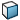Select faces for the plot Select the faces to view results.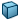Select bodies for the plot Select the bodies to view results.
Display symmetric results

Available for models with symmetry restraints or cyclic symmetry.

Select to view the strain values for the whole model. The results are mirrored about the planes of symmetry for models where you analyze half, a quarter, or one-eighth of the actual geometry.

For cyclic symmetry, results are repeated cyclically about the defined axis of revolution.

Node Values Generates nodal strain plots. Linear interpolation is used to generate a smooth plot.
Element Values Generates elemental strain plots (one value/color for each element).

Plot Step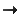Plot for Single Step Select this option to plot the results at the selected solution step.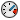Sets the time (in seconds) at which the strain results are plotted. If there are no results available for the requested time, results are plotted for the nearest available solution step number.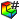Plot Step Sets the solution step number for the plot.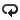Plot Bounds across All Steps Select this option to plot extreme values regardless of the solution step they occur at. Select one of the following:
 Maximum Displays the maximum algebraic values (envelope plot) across all solution steps. Minimum Displays the minimum algebraic values (envelope plot) across all solution steps. Absolute maximum Displays the absolute maximum values (envelope plot) across all solution steps.

Deformed Shape

If checked, the selected component is plotted on the deformed shape of the model.

 Automatic Displays the default Scale Factor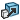that the program uses to scale the largest deformation to 10% of the largest dimension of the smallest box that surrounds the model. True scale Displays the actual deformed shape of the model (Scale Factor is 1.0). User defined Allows you to enter your own Scale Factor. In most cases, a larger scale factor helps to appreciate the deflection.

Property

 Include title text Type a custom title for the plot. Associate plot with name view orientation Associate a predefined view orientation with the active plot.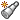Associate with current view Associates the current view with the active plot.